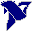# LabVIEW

cancel
Showing results for
Search instead for
Did you mean:

Solved!
Go to solution

## How to calculate the equation from dataset using "Fitting VI's" (polynomial or nonlinear Fit) ?

I have the datasheet from a NTC (Negative Thermistor Coefficient) and I want to calculate the most approximate equation

that describes it's  behaviour. The table has temperature and resistance values. So the resistance of the NTC varies depending on the temperature it detects. I've already tried the next:

- Excel: polynomial fitting

- Maple: polynomial fitting

- LabVIEW:  Polynomial Fit.vi

I've been getting equations with a not very good approximate parameteres. I'm suspecting that this method "polynomial FIT" it's not the best method for this type of data/equation (being a nonlinear equation).

So has someone used the Nonlinear Fit.vi to approximate the behaviour of a dataset? If so, can you explain me how this VI works... I would really appreciate it.

Thanks in advance and I'll wait for your answer.

Message 1 of 25
(2,343 Views)

## Re: How to calculate the equation from dataset using "Fitting VI's" (polynomial or nonlinear Fit) ?

I have not looked at your VI.

Any fitting process needs to start with an appropriate function. To get that you need to look at the physics of your system - in this case a thremistor. A simple search will show that the resistance-temperature relationship is not at all a polynomial. Specifically, the Steinhart-Hart equation is often used.

You may be able to fit the 3 parameters of that equation to your data table using Lev-Mar or something similar.

Lynn

Message 2 of 25
(2,338 Views)

## Re: How to calculate the equation from dataset using "Fitting VI's" (polynomial or nonlinear Fit) ?

Hi JLuna,

could you convert your VI to LV2014 and attach this version too? (This would allow me and others to actully examine your VI.)

Another approach, most often very easy with good results:

Put the resistance-temperature pairs into an array of points. Then use Interpolate1DArray to get the temperature from resistance reading:(Replace my dummy data with you real data!)

One more approach: with NTC thermistors you usually get R0 and B values from datasheet. Then apply the formulas described at Wikipedia!

Best regards,
GerdWusing LV2011SP1 + LV2017 (+LV2020 sometimes) on Win10+cRIO
Message 3 of 25
(2,318 Views)

## Re: How to calculate the equation from dataset using "Fitting VI's" (polynomial or nonlinear Fit) ?

i've just read about this equation. I didn't have notion of it's existance. Apparently it has 3 coefficients that can be

calculated. In your post you mention:

"You may be able to fil 3 parameters of that equation to your data table using Lev-Mar or something similar".

And by "3 parameters" you mean, the coefficients that are needed to be calculated. If I'm interpretating this right, there

is a Nonlinear vi, that uses the method Lev-Mar. In this VI, it has an input called "initial parameters": Is this input referring to this parameters?

**I've never used this type of VI's so I'm having lots of questions...

Thanks for your answer!!

Message 4 of 25
(2,315 Views)

## Re: How to calculate the equation from dataset using "Fitting VI's" (polynomial or nonlinear Fit) ?

Here is the 2014 version. I'll try that interpolating also... I'm a bit skeptical, because the "Interpolate 1D array"mentions to linearize the "y"values from your "x" values. But I'll give that a try also.

I just want to calculate en equation with a very low error. I dunno maybe at most, 2% error.

Thanks for your answer.

Message 5 of 25
(2,311 Views)

## Re: How to calculate the equation from dataset using "Fitting VI's" (polynomial or nonlinear Fit) ?

A higher order polynomial is a nonlinear fit. If you use more normal fit option (just delete these misquided "bisquare" and "SVD for rank defficient ..." inputs!) and things look much better!LabVIEW Champion. It all comes together inGCentralWhat does "Engineering Redefined" mean??
Message 6 of 25
(2,307 Views)

## Re: How to calculate the equation from dataset using "Fitting VI's" (polynomial or nonlinear Fit) ?

@altenbach wrote:

A higher order polynomial is a nonlinear fit. If you use more normal fit option (just delete these misquided "bisquare" and "SVD for rank defficient ..." inputs!) and things look much better!

Here is a 6th order polynomial fit to the two datasets leaving the optional inputs disconnected. Seems good enough.LabVIEW Champion. It all comes together inGCentralWhat does "Engineering Redefined" mean??
Message 7 of 25
(2,305 Views)

## Re: How to calculate the equation from dataset using "Fitting VI's" (polynomial or nonlinear Fit) ?

But yes, if there is a suitable mathematical model with fewer paramters, you should use nonlinear fit.LabVIEW Champion. It all comes together inGCentralWhat does "Engineering Redefined" mean??
Message 8 of 25
(2,301 Views)

## Re: How to calculate the equation from dataset using "Fitting VI's" (polynomial or nonlinear Fit) ?

Can you display the six order equation for those two graphics.

I generated several equations using "Polynomial Fit" and using the calculated equations I substitute a new value of my choice, for

example 23.2. And watched how this equation delivered a similar value to the graphic. So, can you display the equations please?

Message 9 of 25
(2,292 Views)

## Re: How to calculate the equation from dataset using "Fitting VI's" (polynomial or nonlinear Fit) ?

The equations are polynomials and the coefficients I found were:

32080.3
-1484.52
48.3516
-1.35193
0.0224839
-0.000180263
5.39565E-7

and for the lower set:

32080.3
-1484.52
48.3516
-1.35193
0.0224839
-0.000180263
5.39565E-7

Just put them into a DBL array and use polynomial evaluation to calculate the value for any desired x or array of x's.LabVIEW Champion. It all comes together inGCentralWhat does "Engineering Redefined" mean??
Message 10 of 25
(2,290 Views)